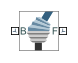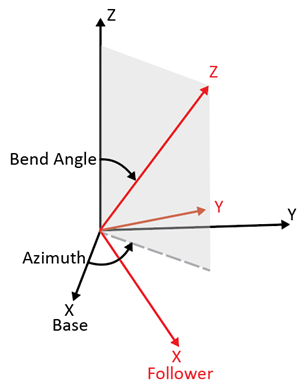# Constant Velocity Joint

Joint that enforces a constant-velocity kinematic constraint between two shafts

• Library:
• Simscape / Multibody / Joints

•## Description

The Constant Velocity Joint block enforces a constant-velocity (CV) kinematic constraint between its base and follower frames, whose origins are coincident throughout the simulation. Specifically, if the Z-axes of the base and follower frames are both fixed with respect to a common reference frame, the Z-components of the two frames' angular velocities with respect to the common reference frame are equal.

The block has two degrees of freedom that allow the Z-axes of the base and follower frames to be arbitrarily oriented relative to each other. The figure shows an example.The black and red frames indicate the base and follower frames of the block. The azimuth rotation about the Z-axis of the base frame locates the plane in which the bend angle occurs. The bend angle about the resulting Y-axis, the red Y-axis, specifies the orientation of the Z-axis of the follower frame with respect to the Z-axis of the base frame.

The block has two parameterizations, ```Rotation Sequence (faster simulation)``` or ```Quaternion (allows zero bend angle)```, to specify the internal states of the joint. Use the rotation-sequence parameterization whenever possible because a simulation with this parameterization is generally faster than a simulation with the quaternion parameterization. See Internal State for more information.

You can specify the desired initial states of the joint with the parameters under State Targets, such as the position and velocity of the azimuth and bend angle.

The block has a variety of sensing abilities. You can sense the azimuth, bend angle, and their time derivatives throughout the simulation. Furthermore, you can sense the forces and torques that act in the joint, such as constraint forces and total torque. See Composite Force/Torque Sensing for more information.

## Ports

### Frame

expand all

Port associated with the base frame of the joint block. In typical applications where a CV joint couples two shafts, the Z-axis of base frame is aligned with the driving shaft.

Port associated with the follower frame of the joint block. In typical applications where a CV joint couples two shafts, the Z-axis of follower frame is aligned with the driven shaft.

### Input

expand all

Input signal that controls the joint mode, specified as a unitless scalar. The joint mode is normal when the input signal is 0 and disengaged when the input signal is -1. The joint mode can be changed many times during a simulation.

#### Dependencies

To enable this port, under Mode Configuration, set Mode to `Provided by Input`.

### Output

expand all

Bend angle of the CV joint, returned as a scalar. This quantity is the angle between the Z-axes of the base and follower frames of the block.

#### Dependencies

To enable this port, under Constant Velocity Primitive (CV) > Sensing > Bend Angle, select Position.

Velocity of the bend angle of the CV joint, returned as a scalar. This quantity equals the time derivative of the signal output from port qb.

#### Dependencies

To enable this port, under Constant Velocity Primitive (CV) > Sensing > Bend Angle, select Velocity.

Acceleration of the bend angle of the CV joint, returned as a scalar. This quantity equals the second time derivative of the signal output from port qb.

#### Dependencies

To enable this port, under Constant Velocity Primitive (CV) > Sensing > Bend Angle, select Acceleration.

Azimuth of the CV joint, returned as a scalar. This quantity is the angle of the rotation about the Z-axis of the base frame. The rotation locates the plane in which the bend angle occurs.

#### Dependencies

To enable this port, under Constant Velocity Primitive (CV) > Sensing > Azimuth, select Position.

Velocity of the azimuth of the CV joint, returned as a scalar. This quantity equals the time derivative of the signal output from port qa.

#### Dependencies

To enable this port, under Constant Velocity Primitive (CV) > Sensing > Azimuth, select Velocity.

Acceleration of the azimuth of the CV joint, returned as a scalar. This quantity equals the second time derivative of the signal output from port qa.

#### Dependencies

To enable this port, under Constant Velocity Primitive (CV) > Sensing > Azimuth, select Acceleration.

Constraint force that acts in the joint, returned as a scalar. The force maintains the translational constraints of the joint. See Sense Constraint Forces for more information.

#### Dependencies

To enable this port, under Composite Force/Torque Sensing, select Constraint Force.

Constraint torque acting in the joint, returned as a scalar. The torque maintains the rotational constraints of the joint. See Force and Torque Sensing for more information.

#### Dependencies

To enable this port, under Composite Force/Torque Sensing, select Constraint Torque.

Total force acting in the joint, returned as a scalar. The total force is the sum of forces transmitted from one frame to the other through the joint. The force includes actuation, internal, and constraint forces. See Force and Torque Sensing for more information.

#### Dependencies

To enable this port, under Composite Force/Torque Sensing, select Total Force.

Total torque acting in the joint, returned as a scalar. The total torque is the sum of torques transmitted from one frame to the other through the joint. The torque includes actuation, internal, and constraint torques. See Force and Torque Sensing for more information.

#### Dependencies

To enable this port, under Composite Force/Torque Sensing, select Total Torque.

## Parameters

expand all

### Constant Velocity Primitive (CV)

Choose the parameterization to specify the internal states of the joint.

```Rotation Sequence (faster simulations)```

The Constant Velocity Joint block uses the Z-Y-Z rotation sequence parameterization. The angle of the second Z-axis rotation is the negative of the angle of the first Z-axis rotation.

Use this method whenever possible because a simulation with this parameterization is generally faster than a simulation with the quaternion parameterization. However, the Z-Y-Z rotation sequence parameterization has a kinematic singularity when the bend angle is zero. If zero bend angle is required, set Internal State to ```Quaternion (allows zero bend angle)```.

```Quaternion (allows zero bend angle)```

The Constant Velocity Joint block uses the quaternion parameterization. To enforce the CV kinematic constraint, the fourth component of the quaternion, the Z-component of the vector part, is always zero.

Use this method when zero bend angle is required. This method does not have a kinematic singularity at zero bend angle but has a kinematic singularity when the bend angle is 180 degrees. A simulation with this parameterization is generally slower than a simulation with the Z-Y-Z parameterization.

Select this parameter to enable parameters for specifying the position target of the joint.

Set the priority level of the position target. See Guiding Assembly for more information.

#### Dependencies

To enable this parameter, under Constant Velocity Primitive (CV) > State Targets, select Specify Position Target.

Whether to specify the position target of the CV joint at the start of simulation using only the bend angle or the bend angle and the azimuth.

#### Dependencies

To enable this parameter, under Constant Velocity Primitive (CV) > State Targets, select Specify Position Target.

Specify the bend angle of the CV joint.

#### Dependencies

To enable this parameter, under Constant Velocity Primitive (CV) > State Targets, select Specify Position Target.

Specify the azimuth of the CV joint.

#### Dependencies

To enable this parameter, under Constant Velocity Primitive (CV) > State Targets > Specify Position Target > Value, select Bend Angle and Azimuth.

Select this parameter to enable parameters for specifying the velocity target of the joint.

Set the priority level of the velocity target. See Guiding Assembly for more information.

#### Dependencies

To enable this parameter, under Constant Velocity Primitive (CV) > State Targets, select Specify Velocity Target.

Whether to specify the velocity target of the CV joint at the start of simulation using only the bend angle or the bend angle and the azimuth.

#### Dependencies

To enable this parameter, under Constant Velocity Primitive (CV) > State Targets, select Specify Velocity Target.

Specify the bend angle velocity of the CV joint.

#### Dependencies

To enable this parameter, under Constant Velocity Primitive (CV) > State Targets, select Specify Velocity Target.

Specify the azimuth velocity of the CV joint.

#### Dependencies

To enable this parameter, under Constant Velocity Primitive (CV) > State Targets > Specify Velocity Target > Value, select Bend Angle and Azimuth.

Select this parameter to enable the port qb.

Select this parameter to enable the port wb.

Select this parameter to enable the port bb.

Select this parameter to enable the port qa.

Select this parameter to enable the port wa.

Select this parameter to enable the port ba.

### Mode Configuration

Specify the joint mode for the simulation.

MethodDescription
`Normal`The joint behaves normally throughout the simulation.
`Disengaged`The joint is disengaged throughout the simulation.
`Provided by Input`This option exposes the mode port that you can connect to an input signal to change the joint mode during the simulation. The joint mode is normal when the input signal is 0 and disengaged when the input signal is -1. The joint mode can be changed many times during the simulation.

### Composite Force/Torque Sensing

Measurement direction, specified as ```Follower on Base``` or ```Base on Follower```.

• `Follower on Base` － Sense the force and torque that the follower frame exerts on the base frame.

• `Base on Follower` － Sense the force and torque that the base frame exerts on the follower frame.

Reversing the direction changes the sign of the measurements. For more information see Force and Torque Measurement Direction.

Frame used to resolve the measurements, specified as `Base` or `Follower`.

• `Base` － The joint block resolves the measurements in the coordinates of the base frame.

• `Follower` － The joint block resolves the measurements in the coordinates of the follower frame.

Select this parameter to enable the port fc.

Select this parameter to enable the port tc.

Select this parameter to enable the port ft.

Select this parameter to enable the port tt.

## Version History

Introduced in R2015a